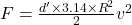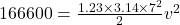## The 12 Mg helicopter is hovering over a lake as the suspended bucket collects 5 m3 of water used to extinguish a fire. Determine the power r

Question

The 12 Mg helicopter is hovering over a lake as the suspended bucket collects 5 m3 of water used to extinguish a fire. Determine the power required by the engine to hold the filled water bucket over the lake. The horizontal blade has a diameter of 14 m. Use rhow = 1000 kg/m3 and rhoa = 1.23 kg/m3

in progress 0
2 months 2021-08-06T05:31:16+00:00 1 Answers 0 views 0

7 MW

Explanation:

mass of helicopter, m = 12 Mg = 12000

volume of water in bucket, V = 5 m³

diameter of blade, D = 14 m

density of water, d = 1000 kg/m³

density of air, d’ = 1.23 kg/m³

The weight of water and the helicopter is balanced by the thrust produced by the rotor blade of the helicopter.

So, force

F = m x g  + V x d x g

F = 12000 x 9.8 + 5 x 1000 x 9.8

F = 166600 N

Let initially the velocity of blade is vv = 41.96 m/

Power of the helicopter, P = Force x velocity

P = 166600 x 41.96

P = 7 x 10^6 Watt

P = 7 MW